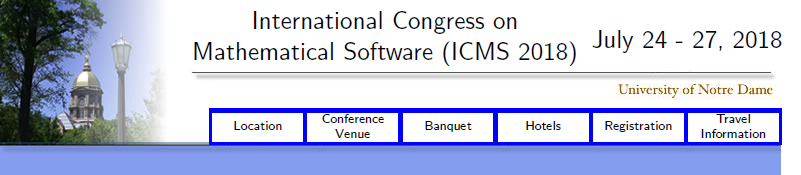International Congress on Mathematical Software  -  ICMS 2018

Conference Photo     Participants at Notre Dame Stadium

Mathematics has a wide variety of branches, from Algebra to Analysis, from Geometry to Number Theory, and many more. One theme across all these branches is the notion of effectivity: mathematical theories often predict the existence of objects with certain properties and it might be important to find such objects. Conversely, to formulate conjectures and new mathematical theories, we may need to explore the space of such objects, and use them to prove new theorems. Mathematical software is the common tool in such quests.

Mathematics also has increasing overlap with many mathematical disciplines such as Computer Science and the emerging area of Computational Sciences and Engineering. A key factor in this convergence of mathematical disciplines is the idea of computation, as manifested in the form of mathematical software in such disciplines.

We in the International Conference of Mathematical Software believe that the appearance of mathematical software is one of the most important new development in mathematics, and this phenomena should be studied as a coherent whole. We hope this conference can serve as the main forum for mathematicians, scientists and programmers who are interested in development of mathematical software.

Acknowledgements:

We gratefully acknowledge the support of Vice President for Research, College of Science, and Department of Applied and Computational Mathematics and Statistics at the University of Notre Dame, the National Science Foundation, and Oak Ridge Associated Universities (ORAU).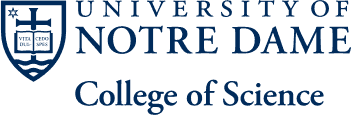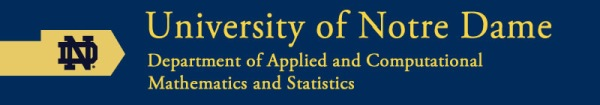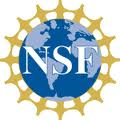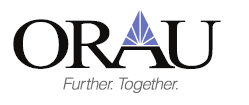Department of Applied and Computational Mathematics and Statistics
153 Hurley Hall | Notre Dame, IN 46556
Office: (574) 631-8630 | acms@nd.edu